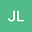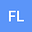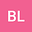loading page

Fujita blow-up phenomena of solutions for a Dirichlet problem of parabolic equations with space-time coefficients
•••• Jiaqi Liu,
• Fengjie Li,
• Bingchen Liu
Jiaqi Liu
China University of Petroleum Huadong
Author ProfileFengjie Li
China University of Petroleum Huadong
Author ProfileBingchen Liu
College of Science, China University of Petroleum
Author Profile## Abstract

This paper deals with a homogeneous Dirichlet initial-boundary problem of parabolic equations with different space-time coefficients, $$u_t =\Delta u + t^{\sigma_1} u^{\alpha} + \langle x\rangle^{n} v^{p},\quad v_t =\Delta v + \langle x\rangle^{m} u^{q} + t^{\sigma_2} v^{\beta},$$ where the eight exponents are nonnegative constants and $\langle x\rangle$ is the Japanese brackets. We obtain the Fujita exponents of solutions, which are determined by the eight exponents and the dimension of the space domain. Moreover, simultaneous or non-simultaneous blow-up of the two components of blow-up solutions is discussed with or without conditions on the initial data.

#### Peer review status:UNDER REVIEW

12 May 2021Submitted to Mathematical Methods in the Applied Sciences
12 May 2021Assigned to Editor
12 May 2021Submission Checks Completed
17 May 2021Reviewer(s) Assigned